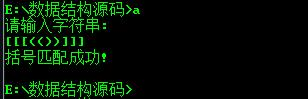• 用的CB 结果不对 代码哪里有问题呢 <p>#include<stdio.h> #include<stdlib.h> int item; <p>struct node{<!-- --> char bracket; struct node* next;...<p>}</p>
• 利用实现括号匹配会非常简单，只需要设置一个，指向栈顶的top；利用top++或top–实现入栈和出栈。 实现匹配还需要条件判断，当出现右括号时候，就与栈顶元素比较。 #include <stdio.h> #include <...
栈是一种只允许在栈顶增加和删除元素的数据结构。
利用栈实现括号匹配会非常简单，只需要设置一个栈，指向栈顶的top；利用top++或top–实现入栈和出栈。
实现匹配还需要条件判断，当出现右括号时候，就与栈顶元素比较。

#include <stdio.h>
#include <stdlib.h>
#include <string.h>
int main()
{
//s字符串作栈，top为栈顶，a用来存储所要判断的字符串
char s,a;
gets(a);
int len=strlen(a),i;
int top=0;
for(i=0;i<len;i++)
{
//判断是否是右括号，如果不是将字符入栈
if(a[i]!= ‘)’ && a[i]!=’]’ && a[i]!=’}’)
{
s[++top]=a[i];
}
else
{
//如果是右括号，与栈顶元素比较，匹配则出栈
//判断是否是匹配，只需看top最后的值是否是0，为0则说明括号是匹配的
switch(a[i])
{
case ‘)’:
if(s[top]’(’)
{
--top;
break;
}
else
break;                case ‘]’:
if(s[top]’[’)
{
--top;
break;
}                    else
break;                case ‘}’:
if(s[top]’{’)
{
--top;
break;
}                    else
break;
}
}
}
printf("%d\n",top);
if(top0)
printf(“YES!”);
else
printf(“NO!”);    return 0;
}




展开全文啊哈算法
• 在文字处理软件或编译程序设计时，常常需要检查一个字符串或一个表达式中的括号是否相匹配? 匹配思想：从左至右扫描一个字符串(或表达式)，则每个右括号将与最近遇到的那个左括号匹配。则可以在从左至右扫描过程...
在文字处理软件或编译程序设计时，常常需要检查一个字符串或一个表达式中的括号是否相匹配?
匹配思想：从左至右扫描一个字符串(或表达式)，则每个右括号将与最近遇到的那个左括号相匹配。则可以在从左至右扫描过程中把所遇到的左括号存放到堆栈中。每当遇到一个右括号时，就将它与栈顶的左括号(如果存在)相匹配，同时从栈顶删除该左括号。
算法思想：设置一个栈，当读到左括号时，左括号进栈。当读到右括号时，则从栈中弹出一个元素，与读到的左括号进行匹配，若匹配成功，继续读入；否则匹配失败，返回FLASE。另外,在算法的开始和结束时,栈都应该是空的.所以匹配到最后还要判断栈是否为空,若非空,则说明匹配失败.

代码实现:
采用前面的顺序栈结构来实现此题.

#include"sp_stack.c"

/* 输入括号匹配,其他字符不匹配
* 以空格结束输入
* 匹配错误时直接退出
* 匹配成功,返回true
* 否则返回false
*/

int match(char input[])
{
char ch;
char *str;
int result,i = 0;
scanf("%c",&ch);
/* 创建一个空栈 */
sp_stack s = (sp_stack)malloc(sizeof(stack));
s = stack_init(s);
while(ch != ' ')
{
input[i++] = ch;
/* 判断输入字符是否为括号{}, (), [] */
if ( (ch == '{') || (ch == '(') || (ch == '['))
{
push(s, ch);
get_top(s, str);
}
if (ch == '}' || ch == ']' || ch == ')')
{
pop(s, str);
if (*str == ch)
{
result = true;
scanf("%c", &ch);
continue;
}
else
return false;
}
scanf("%c", &ch);
}
if (stack_length(s) > 0)
result = false;
stack_destory(s);
return result;
}

int main()
{
int i = 0;
char input = {'0'};
if(match(input) == true)
{
printf("匹配成功\n");
}
else
printf("匹配失败\n");
printf("输入的字符串为:\n");
for (i = 0; i < 100; i ++)
{
if(input[i])
{
printf("%c", input[i]);
}
}
printf("\n");
return 0;
}
展开全文• if(db(e)+db(ch)==5){//详情看db函数，符号匹配则相加为5 Pop(L,e); }else{ Push(L,ch); } } scanf("%c",&ch); } if(L.base==L.top){ printf("This Symbol Mathing Victory !\n"); }else...
#include<stdio.h>
#include<stdlib.h>
#include<malloc.h>
typedef struct{
char *top;
char *base;
int stacksize;

}SqStack;
int InitStack(SqStack &L){
L.base=(char *)malloc(100*sizeof(char ));
if(!L.base){
return -1;
}
L.top=L.base;
L.stacksize=100;
return 1;
}
int GetTop(SqStack L,char &e){
if(L.base==L.top){
return -1;
}
e=*(L.top-1);
return 1;
}
int Push(SqStack &L,char e){
if(L.top-L.base>=L.stacksize){
L.base=(char *)realloc(L.base,(100+10)*sizeof(char ));
if(!L.base){
return -1;
}
L.top+=L.stacksize;
L.stacksize+=10;
}

*(L.top++)=e;
return 1;
}
int Pop(SqStack &L,char &e){
if(L.top==L.base){
return -1;
}
e=*(--L.top);
return 1;
}
int db(char a){
switch(a){
case '[':
return 1;
case '(':
return 2;
case ')':
return 3;
case ']':
return 4;
}
return 0;
}
void symbolMathing(){
SqStack L;
char ch;
char e;
InitStack(L);
scanf("%c",&ch);
while(ch!='\n'){
if(L.base==L.top){
Push(L,ch);
}else{
GetTop(L,e);
if(db(e)+db(ch)==5){//详情看db函数，符号匹配则相加为5
Pop(L,e);
}else{
Push(L,ch);
}
}
scanf("%c",&ch);
}
if(L.base==L.top){
printf("This Symbol Mathing Victory !\n");
}else{
printf("This Symbol Mathing Fail!\n");
}
}
void main(){
symbolMathing();
}



展开全文• 数据结构之---C语言实现括号匹配实现）
#include <stdio.h>
#include <stdlib.h>
#define STACKINCREAMENT 10
#define STACK_INIT_SIZE 100
#define OVERFLOW -2
#define OK 1
#define ERROR 0
typedef int status;
typedef char SElemtype;
typedef struct
{
SElemtype *base;
SElemtype *top;
status stacksize;
}sqstack;

//初始化栈
status Init(sqstack *s)
{
s->base = (SElemtype *)malloc(STACK_INIT_SIZE * sizeof(SElemtype));
if(!s->base)
exit(OVERFLOW);
s->top = s->base;
s->stacksize = STACK_INIT_SIZE ;
return OK;
}

//获取栈顶元素
status Gettop(sqstack *s, SElemtype e)
{
if(s->top == s->base)
return ERROR;
e = *(s->top-1);
return OK;
}

//压栈
status push(sqstack *s, SElemtype e)
{
if(s->top - s->base >= s->stacksize)
{
s->base = (SElemtype *)realloc(s->base, (s->stacksize + STACKINCREAMENT) * sizeof(SElemtype));
if(!s->base)
exit(OVERFLOW);
s->top = s->base + s->stacksize;
s->stacksize += STACKINCREAMENT;
}
*s->top++ = e;
return OK;
}

//出栈
status pop(sqstack *s, SElemtype *e)
{
if(s->top == s->base)
return ERROR;
*e=*--s->top;
return OK;
}

//判断是否为空栈
status stackempty(sqstack *s)
{
if(s->top==s->base)
return OK;
return ERROR;
}

//清空栈
status clearstack(sqstack *s)
{
if(s->top == s->base)
return ERROR;
s->top = s->base;
return OK;
}

//括号匹配算法
status Parenthesis_match(sqstack *s,char *str)
{
int i=0, flag=0;
SElemtype e;
while(str[i] != '\0')
{
switch(str[i])
{
case '(':
push(s,str[i]);
break;
case '[':
push(s,str[i]);
break;
case ')':
{
pop(s,&e);
if(e != '(')
flag=1;
}
break;
case ']':
{
pop(s,&e);
if(e!='[')
flag=1;
}
break;
default:
break;
}
if(flag)
break;
i++;
}

if(!flag && stackempty(s))
printf("括号匹配成功!\n");
else
printf("括号匹配失败!\n");
return OK;
}

int main()
{
char str, enter;
sqstack s;
Init(&s);
printf("请输入字符串:\n");
scanf("%s",str);
scanf("%c",&enter);
Parenthesis_match(&s,str);
return 0;
}展开全文• 我们在编写程序的时候经常会遇到括号不匹配而导致语法错误的问题，现在我们可以通过来自己实现括号匹配的问题，了解其实现细节。 我们假设在表达式中只有()[]这两种括号，其嵌套的方式是随意的。检验括号是否匹配...
• c语言下，利用的结构，判断一个表达式的括号是否合法
• 基于c语言括号匹配算法算法要求算法思路初始化（将清空）判断是否为空进栈出栈定义主函数完整代码注释 算法要求 完成括号匹配的算法，输入一个字符串，长度不超过100，检查字符串是否可以括号匹配 算法...
• 括号匹配c语言来实现 #include "stdio.h" void init(){ char s; int top; char ch; top=0; printf("请输入符号\n"); ch=getchar(); while(ch!=EOF && ch !='\n'){ switch(ch){ case '(' : s[top+...
• 用一个，就能解决该问题，左括号栈顶字符必须和第一个入栈的右括号字符匹配介绍：是一种特殊的线性表，仅能在线性表的一端操作。 的特性：后进先出（LIFO) 由于正在学数据结构，于是的定义与操作都是...
• c语言栈训练题目:括号匹配 基本思路: 当前字符不是括号，不做处理； 当前字符是左括号，则进栈； 当前字符是右括号：若栈空，则表明该“右括号”多余，不匹 配，结束；否则和栈顶元素比较，若不匹配，结束；若和...算法
• C语言利用的“先进后出”的思想，实现括号匹配的问题。
• 括号匹配检测，对于一串带括号的字符 1.如果是左括号，入栈 2.如果是右括号，与栈顶元素比较， 若形成括号对，则栈顶左括号出栈； 若不能形成括号对，则括号不能匹配 */ # include # include # define ...
• 看到算法书上提到了括号匹配问题，并没有给出解答。就自己摸索写了一下，用的是类似的形式，但还没有用到push和pop，看起来比较好懂一点。 C代码 #include <stdio.h> #include <stdlib.h> #include &...
• 问题描述 已知以字符型顺序表表示的表达式含有三...存储结构：顺序和链式。 当n个元素以某种顺序进栈，并且可在任意时刻出栈时，所获得元素排列的数目恰好满足函数Catalan（）的计算， 即N= 。数据结构
• 判断输入的括号字符串是否匹配括号有()、[]、{}、四种， 匹配的定义如下： (1) 空字符串为匹配。 (2) 若S为匹配字符串，则(S)、[S]、{S}、为匹配自串。 (3) 两个匹配字符串串接亦为匹配字符串。 输入格式: 输入...c
• printf("右括号比左括号多"); return; } else { GetTop(S,x); if(x=='{'&&a[i]=='}'||x=='['&&a[i]==']'||x=='('&&a[i]==')') { Pop(S,x); Pop(S,x); ...
• 二、方便起见，这里我们使用的顺序存储结构来存储待匹配的元素： 因为存储的是括号，所以数据部分是char类型。 我们开辟了10个连续的char型空间存储元素，1个Int型空间存储指针，指针的值为数组下标。 #include<...
• 利用顺序的操作函数 typedef char SElemType; #include"Stack.h" int kuohaopipei() { char p; SqStack s; char temp; InitStack(s); p=getchar(); while(p!='\n') { if(p=='('||p=='['||p=='{') Push(s,...数据结构
• C语言实现括号匹配，以前学的时候一直是用来实现的，最近发现用来实现他真是杀鸡牛刀。
• 例如：{}[()]、{[()]}、()[]{}这种大中小括号成对出现（位置不限）则为括号匹配，反之则不匹配，如{()[ 接下来看一下实现方式 的定义以及相关操作 //的定义 typedef struct{ char elem[stack_size]; int top;...
• /*括号匹配算法*/ #include<stdio.h> #define FALSE 0 #define TRUE 1 #define MAXSIZE 100 typedef char DataType; //定义 typedef struct stack{ DataType data[MAXSIZE]; int top; }SeqStack; ...
• 这天，小特生日。... 1.-对括号代表一个礼物盒子，盒子里面可以再放盒子，即括号里可以再放括号;若-对括号里没有括号，则代表里面放着礼物。 2.单独的一对括号代表这个礼物只有1层包装，价值为1 ...
• 括号配对问题 时间限制：3000 ms | 内存限制：65535 KB 难度：3 描述 现在，有一行括号序列，请你检查这行括号是否配对。 输入 第一行输入一个数N（0 输出 每组输入数据的输出占一行，如果...
• 括号匹配在一个表达式中含有圆括号或方括号等来表示运算的优先级，将这些括号提取出来就构成了括号序列例如：表达式[(A+B)*C]-[E-F] 其括号序列为[()][]合法的括号序列称为匹配序列，不合法的括号序列称为不匹配序列......

# c语言栈括号匹配c语言 订阅